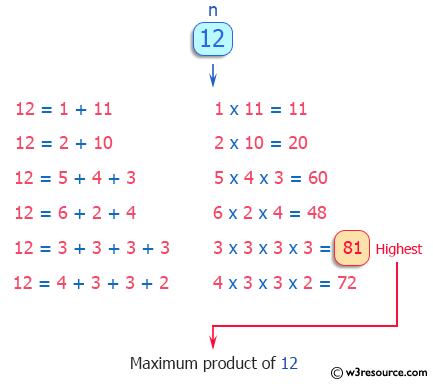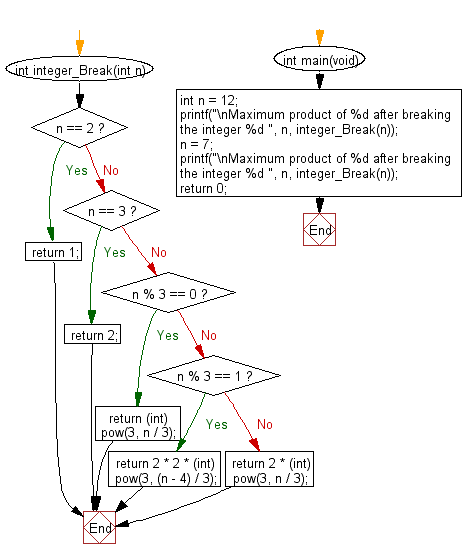﻿ C : Maximum product from an integer breaking the integer

# C Exercises: Get the maximum product from a given integer after breaking the integer into the sum of at least two positive integers

## C Programming Mathematics: Exercise-15 with Solution

Write a C program to get the maximum product of a given integer after breaking the integer into the sum of at least two positive integers.

Example:
Input: 12
Output: 81
Explanation: 12 = 3 + 3 + 3 + 3, 3 x 3 × 3 × 3 = 81.
Input: 7
Output: 12
Explanation: 7 = 3 + 2 + 2, 3 x 2 x 2 = 12.

Pictorial Presentation:Sample Solution:

C Code:

``````#include <stdio.h>
#include <math.h>
#include <stdlib.h>

int integer_Break(int n) {
if (n == 2) {
return 1;
} else if (n == 3) {
return 2;
} else if (n % 3 == 0) {
return (int) pow(3, n / 3);
} else if (n % 3 == 1) {
return 2 * 2 * (int) pow(3, (n - 4) / 3);
} else {
return 2 * (int) pow(3, n / 3);
}
}

int main(void)
{
int n = 12;
printf("\nMaximum product of %d after breaking the integer %d ", n, integer_Break(n));
n = 7;
printf("\nMaximum product of %d after breaking the integer %d ", n, integer_Break(n));
return 0;
}
```
```

Sample Output:

```Maximum product of 12 after breaking the integer 81
Maximum product of 7 after breaking the integer 12
```

Flowchart:C Programming Code Editor:

Improve this sample solution and post your code through Disqus.

What is the difficulty level of this exercise?

Test your Programming skills with w3resource's quiz.

﻿